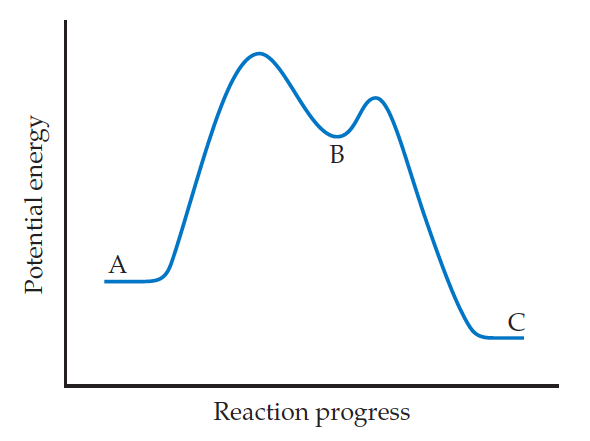Chemistry Practice Problems Energy Diagram Practice Problems Solution: For the reaction A → C, is ΔE positive, negative, ...

⚠️Our tutors found the solution shown to be helpful for the problem you're searching for. We don't have the exact solution yet.

# Solution: For the reaction A → C, is ΔE positive, negative, or zero?

###### Problem

For the reaction A → C, is ΔE positive, negative, or zero?Energy Diagram

Energy Diagram

#### Q. Energy profile for conversion of methyl isonitrile (H3CNC) to its isomer acetonitrile (H3CCN).How does the energy needed to overcome the energy barrie...

Solved • Thu Dec 06 2018 09:35:45 GMT-0500 (EST)

Energy Diagram

#### Q. The following diagram shows the reaction profile of a reaction.Label the components indicated by the boxes.

Solved • Thu Dec 06 2018 09:35:35 GMT-0500 (EST)

Energy Diagram

#### Q. For the elementary process N2O5 (g) → NO2 (g) + NO3 (g) the activation energy (Ea) and overall E are 154 kJ/mol, and 136 kJ/mol, respectively.What is ...

Solved • Thu Dec 06 2018 09:35:35 GMT-0500 (EST)

Energy Diagram

#### Q. The energy of activation for the decomposition of 2 mol of HI to H2 and I2 in the gas phase is 185 kJ. The heat of formation of HI(g) from H2 (g) and ...

Solved • Thu Dec 06 2018 09:35:21 GMT-0500 (EST)